# Show all of your work for full credit. You must give valid reasons to be awarded...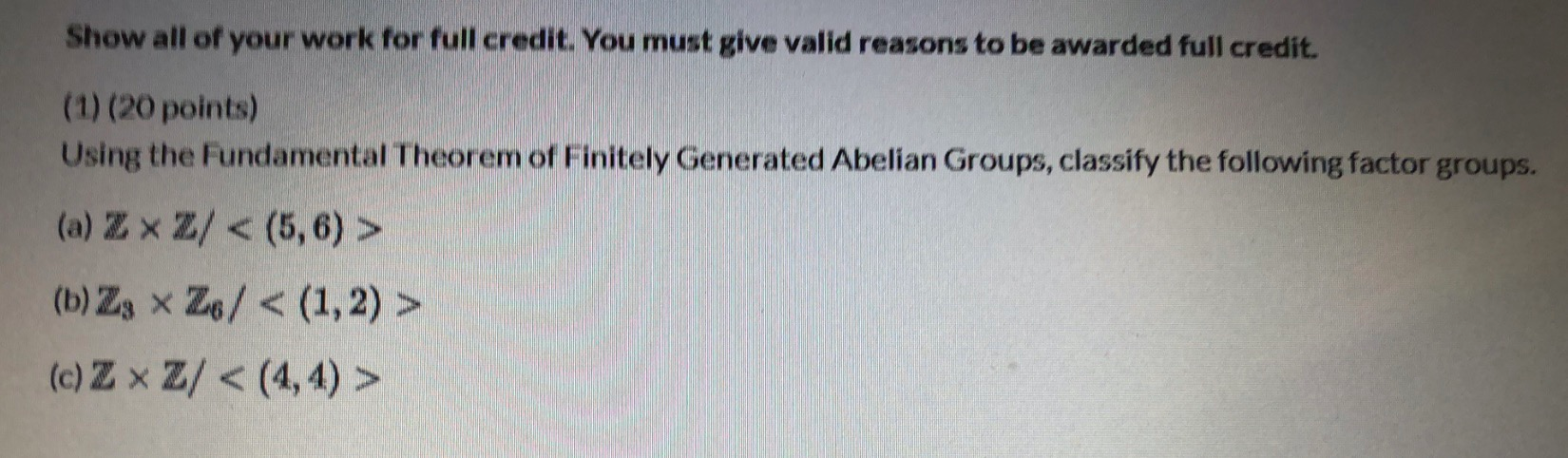Show all of your work for full credit. You must give valid reasons to be awarded full credit. (1) (20 points) Using the Fundamental Theorem of Finitely Generated Abelian Groups, classify the following factor groups. (a) Zx Z/ < (5,6) > (b) Zx x Z6/ < (1,2) > (c) ZxZ/ < (4,4) >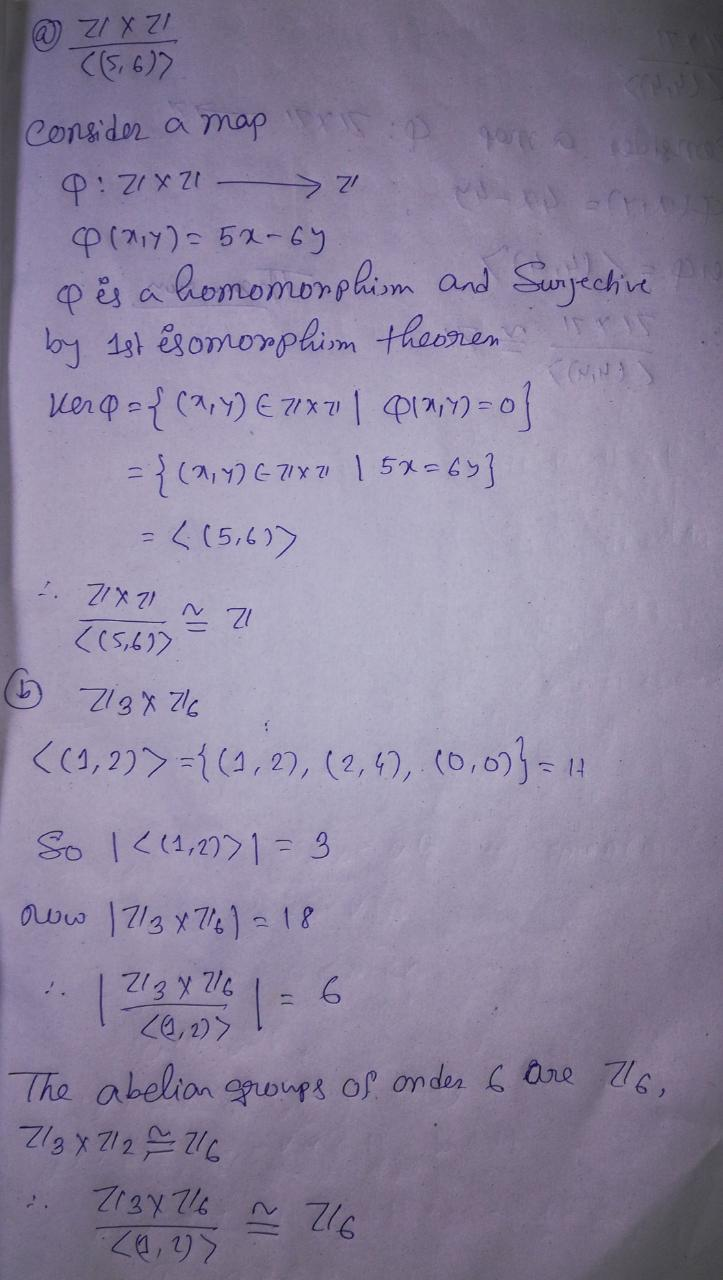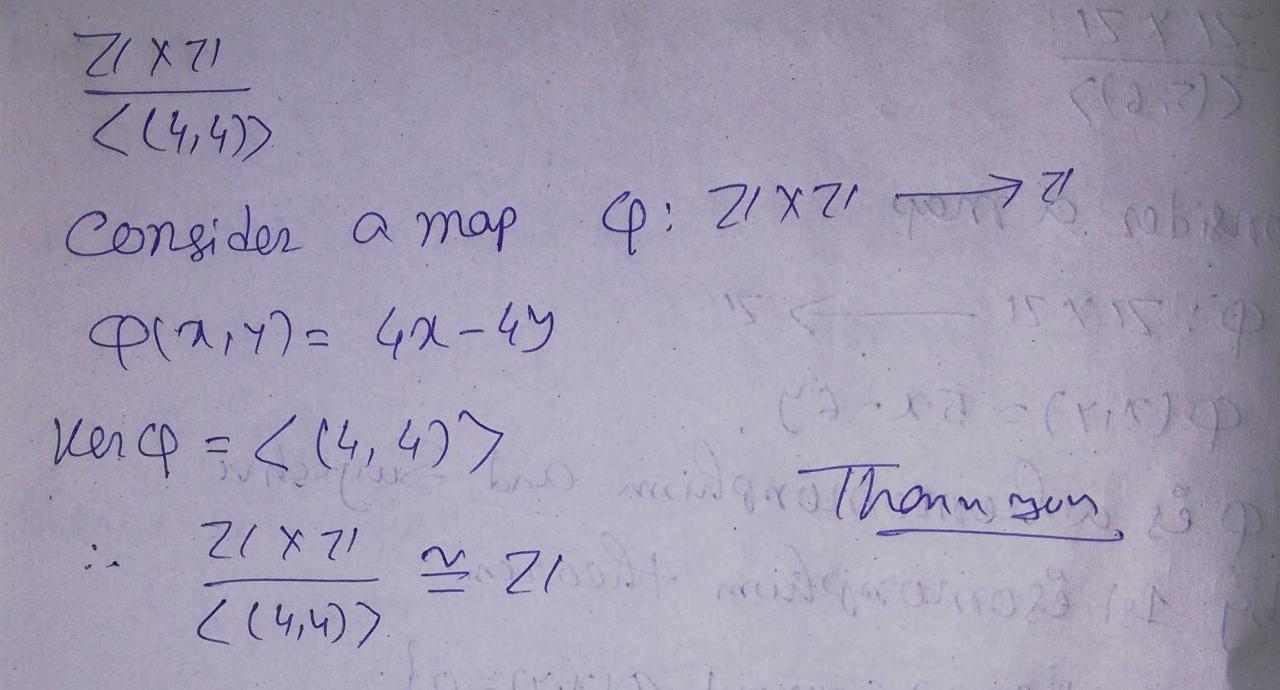##### Add Answer of: Show all of your work for full credit. You must give valid reasons to be awarded...
Similar Homework Help Questions
• ### (1) (20 points) Using the Fundamental Theorem of Finitely Generated Abelian Groups, classify the following factor...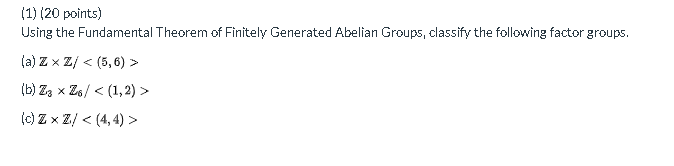(1) (20 points) Using the Fundamental Theorem of Finitely Generated Abelian Groups, classify the following factor groups. (a) ZxZ/ < (5,6) > (b) Zz Z6/ < (1,2) (0) ZxZ/ < (4,4) >

• ### ASAP (1) (20 points) Using the Fundamental Theorem of Finitely Generated Abelian Groups, classify the following...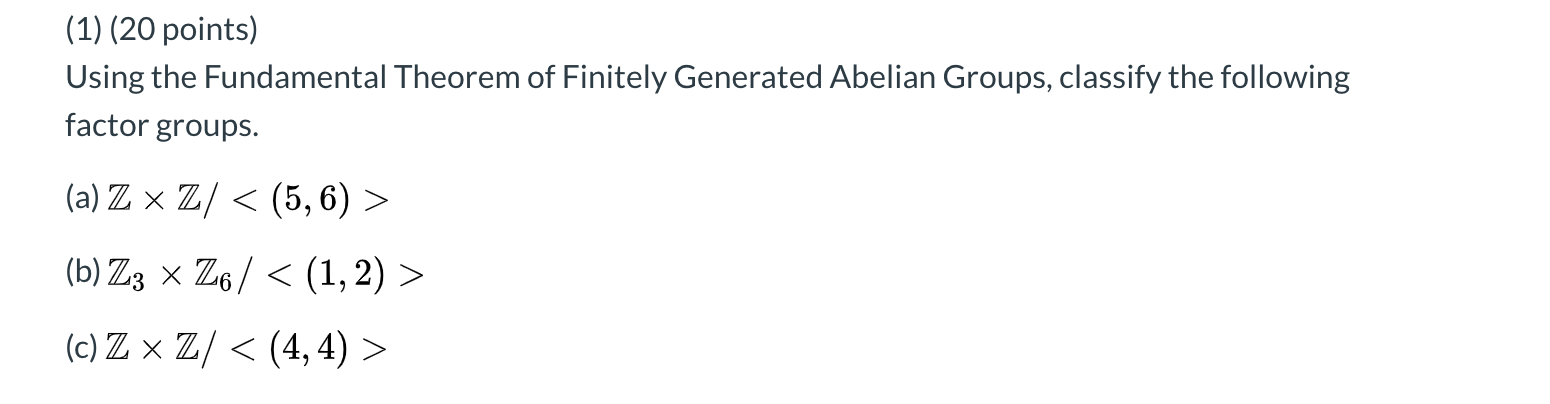ASAP (1) (20 points) Using the Fundamental Theorem of Finitely Generated Abelian Groups, classify the following factor groups. (a) Z x Z/ < (5,6) > (b) Z3 x Z6/ < (1, 2) > (c) Zx Z/ < (4,4) >

• ### Using the Fundamental Theorem of Finitely Generated Abelian Groups, classify the following factor groups. (a) Z...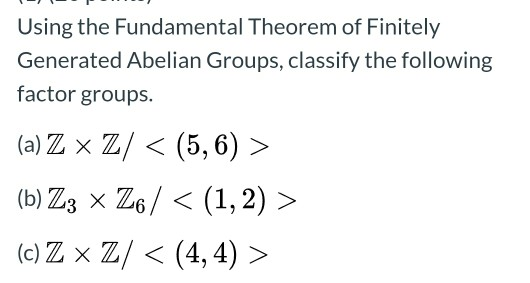Using the Fundamental Theorem of Finitely Generated Abelian Groups, classify the following factor groups. (a) Z x Z/ < (5,6) > (b) Z3 x Z6 / < (1,2) > (c) Z x Z/ < (4,4) >

• ### Need Help ASAP due very soon. Thank You! Using the Fundamental Theorem of Finitely Generated Abelian...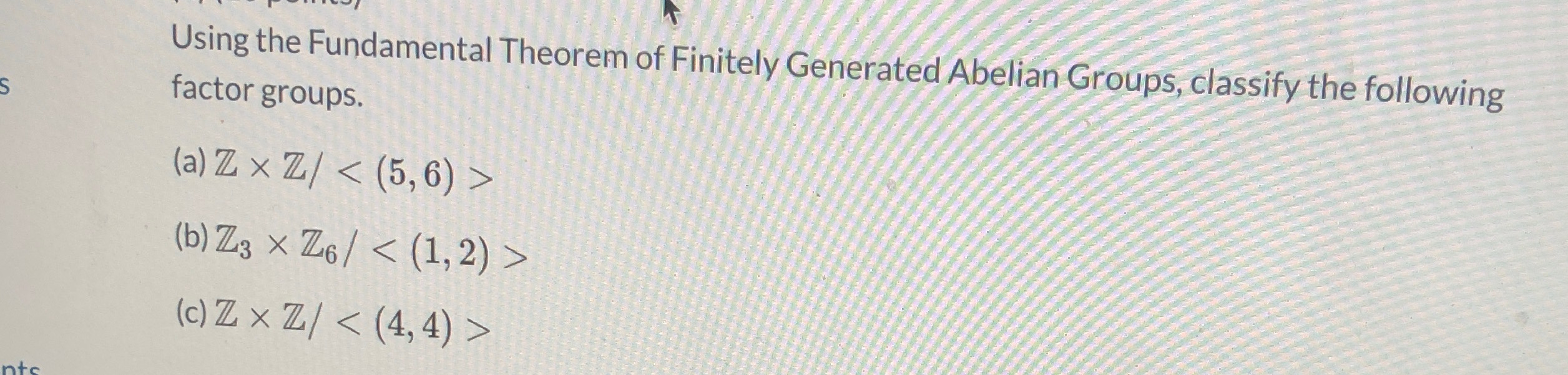Need Help ASAP due very soon. Thank You! Using the Fundamental Theorem of Finitely Generated Abelian Groups, classify the following factor groups. S (a) Z x Z/ < (5,6) > (b) Z3 x Z6/ < (1, 2) > (c) Z x Z/ < (4,4) > nto

• ### (5)(20 points) In the problems below, give the order of the element in the indicated factor...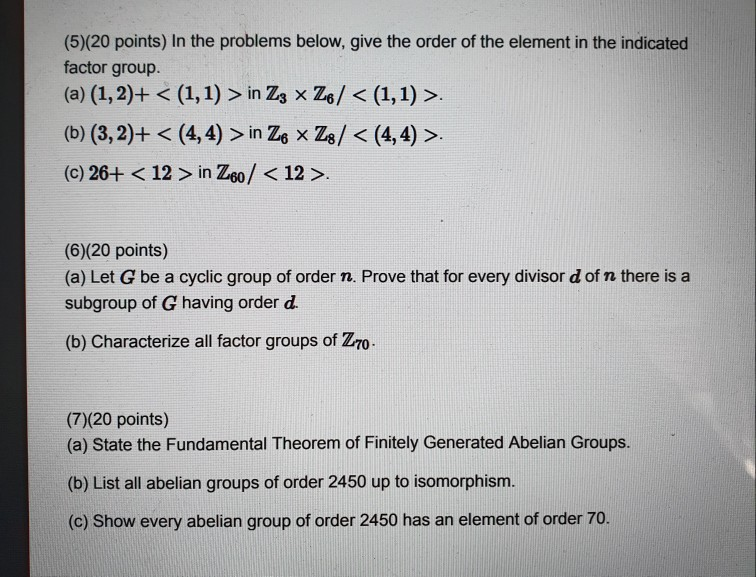(5)(20 points) In the problems below, give the order of the element in the indicated factor group. (a) (1, 2)+ < (1,1) > in Z3 x Z6/ < (1,1) >. (b) (3,2)+ < (4,4) > in Ze * Z8/ < (4,4) >. (c) 26+ < 12 > in Z60 / <12>. (6)(20 points) (a) Let G be a cyclic group of order n. Prove that for every divisor d of n there is a subgroup of G having order d....

• ### (1) (20 points) Using the Fundamental Theorem of Finitely Generated Abelian Groups, classify the following factor...

(1) (20 points) Using the Fundamental Theorem of Finitely Generated Abelian Groups, classify the following factor groups. (a) Z × Z / < ( 5 , 6 ) > (b) Z 3 × Z 6 / < ( 1 , 2 ) > (c) Z x Z / < (4, 4) >

• ### Note: for this problem, you must show all of your work for full credit. 5. A...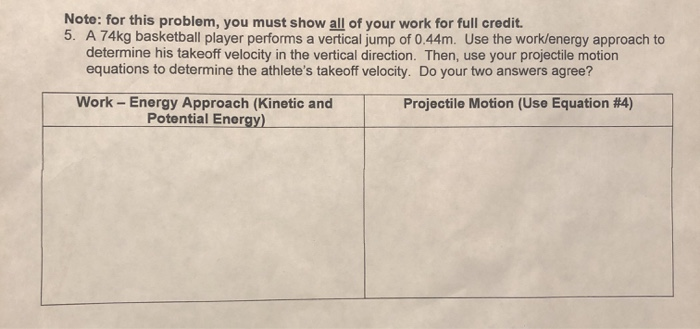Note: for this problem, you must show all of your work for full credit. 5. A 74kg basketball player performs a vertical jump of 0.44m. Use the work/energy approach to determine his takeoff velocity in the vertical direction. Then, use your projectile motion equations to determine the athlete's takeoff velocity. Do your two answers agree? Work - Energy Approach (Kinetic and Potential Energy) Projectile Motion (Use Equation #4)

• ### You must show all your work to receive full credit for each problem. Final answers must...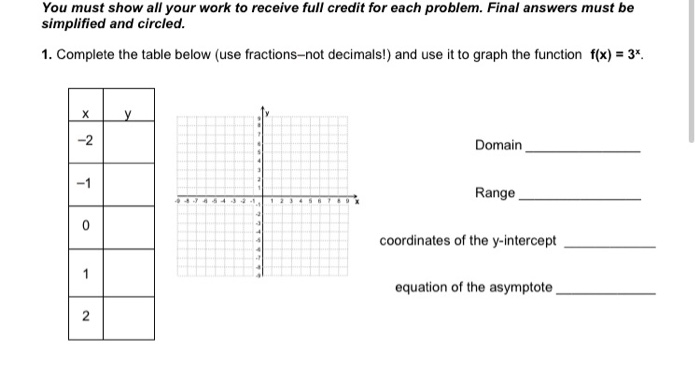You must show all your work to receive full credit for each problem. Final answers must be simplified and circled. 1. Complete the table below (use fractions not decimals!) and use it to graph the function f(x) = 3*. X у -2 Domain -1 Range O coordinates of the y-intercept 1 equation of the asymptote N

• ### Note: You MUST show ALL your work to receive FULL credit- that is, show timelines and...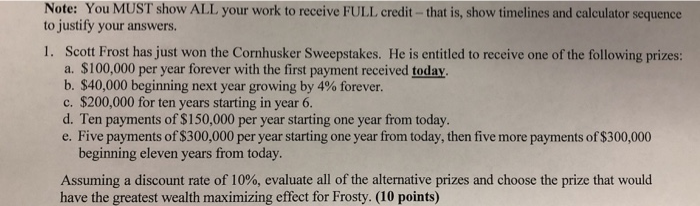Note: You MUST show ALL your work to receive FULL credit- that is, show timelines and calculator sequence to justify your answers. 1. Scott Frost has just won the Cornhusker Sweepstakes. He is entitled to receive one of the following prizes: a. \$100,000 per year forever with the first payment received today b. \$40,000 beginning next year growing by 4% forever. c. \$200,000 for ten years starting in year 6. d. Ten payments of \$150,000 per year starting one year...

• ### You must SHOW ALL OF YOUR WORK, including unit analysis, to receive full credit for each...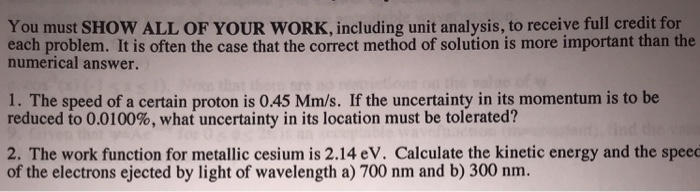You must SHOW ALL OF YOUR WORK, including unit analysis, to receive full credit for each problem. It is often the case that the correct method of solution is more important than the numerical answer. 1. The speed of a certain proton is 045 Mm/s. If the uncertainty in its momentum is to be reduced to 0.0100%, what uncertainty in its location must be tolerated? 2. The work function for metallic cesium is 2.14 eV. Calculate the kinetic energy and...

Free Homework App